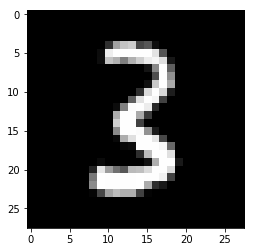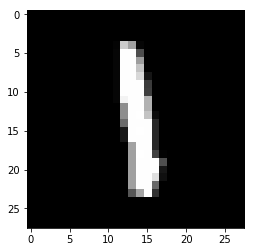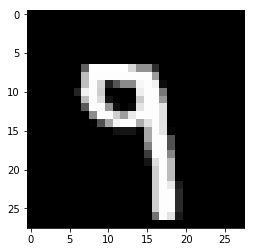#### Note: This is a mirror of the official fast.ai notebook for the DSNet Study Group, please refer the official course repo for the latest notebooks.

In [ ]:
%load_ext autoreload
%autoreload 2

%matplotlib inline

In [ ]:
#export
from exp.nb_02 import *
import torch.nn.functional as F

### Initial setup

#### Data

In [ ]:
mpl.rcParams['image.cmap'] = 'gray'
In [ ]:
x_train,y_train,x_valid,y_valid = get_data()
In [ ]:
n,m = x_train.shape
c = y_train.max()+1
nh = 50
In [ ]:
class Model(nn.Module):
def __init__(self, n_in, nh, n_out):
super().__init__()
self.layers = [nn.Linear(n_in,nh), nn.ReLU(), nn.Linear(nh,n_out)]

def __call__(self, x):
for l in self.layers: x = l(x)
return x
In [ ]:
model = Model(m, nh, 10)
In [ ]:
pred = model(x_train)

#### Cross entropy loss

First, we will need to compute the softmax of our activations. This is defined by:

$\hbox{softmax(x)}_{i} = \frac{e^{x_{i}}}{e^{x_{0}} + e^{x_{1}} + \cdots + e^{x_{n-1}}}$

or more concisely:

$\hbox{softmax(x)}_{i} = \frac{e^{x_{i}}}{\sum_{0 \leq j \leq n-1} e^{x_{j}}}$

In practice, we will need the log of the softmax when we calculate the loss.

In [ ]:
def log_softmax(x): return (x.exp()/(x.exp().sum(-1,keepdim=True))).log()
In [ ]:
sm_pred = log_softmax(pred)

The cross entropy loss for some target $$x$$ and some prediction $$p(x)$$ is given by:

$-\sum x\, \log p(x)$

But since our $$x$$s are 1-hot encoded, this can be rewritten as $$-\log(p_{i})$$ where i is the index of the desired target.

This can be done using numpy-style integer array indexing. Note that PyTorch supports all the tricks in the advanced indexing methods discussed in that link.

In [ ]:
y_train[:3]
Out[]:
tensor([5, 0, 4])
In [ ]:
sm_pred[[0,1,2], [5,0,4]]
Out[]:
tensor([-2.2674, -2.1714, -2.3043], grad_fn=<IndexBackward>)
In [ ]:
y_train.shape
Out[]:
50000
In [ ]:
def nll(input, target): return -input[range(target.shape), target].mean()
In [ ]:
loss = nll(sm_pred, y_train)
In [ ]:
loss
Out[]:
tensor(2.3019, grad_fn=<NegBackward>)

Note that the formula

$\log \left ( \frac{a}{b} \right ) = \log(a) - \log(b)$

gives a simplification when we compute the log softmax, which was previously defined as (x.exp()/(x.exp().sum(-1,keepdim=True))).log()

In [ ]:
def log_softmax(x): return x - x.exp().sum(-1,keepdim=True).log()
In [ ]:
test_near(nll(log_softmax(pred), y_train), loss)

Then, there is a way to compute the log of the sum of exponentials in a more stable way, called the LogSumExp trick. The idea is to use the following formula:

$\log \left ( \sum_{j=1}^{n} e^{x_{j}} \right ) = \log \left ( e^{a} \sum_{j=1}^{n} e^{x_{j}-a} \right ) = a + \log \left ( \sum_{j=1}^{n} e^{x_{j}-a} \right )$

where a is the maximum of the $$x_{j}$$.

In [ ]:
def logsumexp(x):
m = x.max(-1)
return m + (x-m[:,None]).exp().sum(-1).log()

This way, we will avoid an overflow when taking the exponential of a big activation. In PyTorch, this is already implemented for us.

In [ ]:
test_near(logsumexp(pred), pred.logsumexp(-1))

So we can use it for our log_softmax function.

In [ ]:
def log_softmax(x): return x - x.logsumexp(-1,keepdim=True)
In [ ]:
test_near(nll(log_softmax(pred), y_train), loss)

Then use PyTorch's implementation.

In [ ]:
test_near(F.nll_loss(F.log_softmax(pred, -1), y_train), loss)

In PyTorch, F.log_softmax and F.nll_loss are combined in one optimized function, F.cross_entropy.

In [ ]:
test_near(F.cross_entropy(pred, y_train), loss)

### Basic training loop

Basically the training loop repeats over the following steps:

• get the output of the model on a batch of inputs
• compare the output to the labels we have and compute a loss
• calculate the gradients of the loss with respect to every parameter of the model
• update said parameters with those gradients to make them a little bit better
In [ ]:
loss_func = F.cross_entropy
In [ ]:
#export
def accuracy(out, yb): return (torch.argmax(out, dim=1)==yb).float().mean()
In [ ]:
bs=64                  # batch size

xb = x_train[0:bs]     # a mini-batch from x
preds = model(xb)      # predictions
preds, preds.shape
Out[]:
(tensor([ 0.0154,  0.2477, -0.0076, -0.1921, -0.0089,  0.0422,  0.0218, -0.0388,
-0.0303, -0.0328], grad_fn=<SelectBackward>), torch.Size([64, 10]))
In [ ]:
yb = y_train[0:bs]
loss_func(preds, yb)

Out[]:
tensor(2.3076, grad_fn=<NllLossBackward>)
In [ ]:
accuracy(preds, yb)
Out[]:
tensor(0.1719)
In [ ]:
lr = 0.5   # learning rate
epochs = 1 # how many epochs to train for
In [ ]:
for epoch in range(epochs):
for i in range((n-1)//bs + 1):
#         set_trace()
start_i = i*bs
end_i = start_i+bs
xb = x_train[start_i:end_i]
yb = y_train[start_i:end_i]
loss = loss_func(model(xb), yb)

loss.backward()
with torch.no_grad():
for l in model.layers:
if hasattr(l, 'weight'):
l.weight -= l.weight.grad * lr
l.bias   -= l.bias.grad   * lr
l.weight.grad.zero_()
l.bias  .grad.zero_()

In [ ]:
loss_func(model(xb), yb), accuracy(model(xb), yb)
Out[]:
(tensor(0.3465, grad_fn=<NllLossBackward>), tensor(0.9375))

### Using parameters and optim

#### Parameters

Use nn.Module.__setattr__ and move relu to functional:

In [ ]:
class Model(nn.Module):
def __init__(self, n_in, nh, n_out):
super().__init__()
self.l1 = nn.Linear(n_in,nh)
self.l2 = nn.Linear(nh,n_out)

def __call__(self, x): return self.l2(F.relu(self.l1(x)))
In [ ]:
model = Model(m, nh, 10)
In [ ]:
for name,l in model.named_children(): print(f"{name}: {l}")
l1: Linear(in_features=784, out_features=50, bias=True) l2: Linear(in_features=50, out_features=10, bias=True) 
In [ ]:
model
Out[]:
Model(
(l1): Linear(in_features=784, out_features=50, bias=True)
(l2): Linear(in_features=50, out_features=10, bias=True)
)
In [ ]:
model.l1
Out[]:
Linear(in_features=784, out_features=50, bias=True)
In [ ]:
def fit():
for epoch in range(epochs):
for i in range((n-1)//bs + 1):
start_i = i*bs
end_i = start_i+bs
xb = x_train[start_i:end_i]
yb = y_train[start_i:end_i]
loss = loss_func(model(xb), yb)

loss.backward()
with torch.no_grad():
for p in model.parameters(): p -= p.grad * lr
model.zero_grad()

In [ ]:
fit()
loss_func(model(xb), yb), accuracy(model(xb), yb)

Out[]:
(tensor(0.1094, grad_fn=<NllLossBackward>), tensor(0.9375))

Behind the scenes, PyTorch overrides the __setattr__ function in nn.Module so that the submodules you define are properly registered as parameters of the model.

In [ ]:
class DummyModule():
def __init__(self, n_in, nh, n_out):
self._modules = {}
self.l1 = nn.Linear(n_in,nh)
self.l2 = nn.Linear(nh,n_out)

def __setattr__(self,k,v):
if not k.startswith("_"): self._modules[k] = v
super().__setattr__(k,v)

def __repr__(self): return f'{self._modules}'

def parameters(self):
for l in self._modules.values():
for p in l.parameters(): yield p
In [ ]:
mdl = DummyModule(m,nh,10)
mdl

Out[]:
{'l1': Linear(in_features=784, out_features=50, bias=True), 'l2': Linear(in_features=50, out_features=10, bias=True)}
In [ ]:
[o.shape for o in mdl.parameters()]
Out[]:
[torch.Size([50, 784]),
torch.Size(),
torch.Size([10, 50]),
torch.Size()]

#### Registering modules

We can use the original layers approach, but we have to register the modules.

In [ ]:
layers = [nn.Linear(m,nh), nn.ReLU(), nn.Linear(nh,10)]
In [ ]:
class Model(nn.Module):
def __init__(self, layers):
super().__init__()
self.layers = layers
for i,l in enumerate(self.layers): self.add_module(f'layer_{i}', l)

def __call__(self, x):
for l in self.layers: x = l(x)
return x
In [ ]:
model = Model(layers)
In [ ]:
model
Out[]:
Model(
(layer_0): Linear(in_features=784, out_features=50, bias=True)
(layer_1): ReLU()
(layer_2): Linear(in_features=50, out_features=10, bias=True)
)

#### nn.ModuleList

nn.ModuleList does this for us.

In [ ]:
class SequentialModel(nn.Module):
def __init__(self, layers):
super().__init__()
self.layers = nn.ModuleList(layers)

def __call__(self, x):
for l in self.layers: x = l(x)
return x
In [ ]:
model = SequentialModel(layers)
In [ ]:
model
Out[]:
Model(
(layers): ModuleList(
(0): Linear(in_features=784, out_features=50, bias=True)
(1): ReLU()
(2): Linear(in_features=50, out_features=10, bias=True)
)
)
In [ ]:
fit()
loss_func(model(xb), yb), accuracy(model(xb), yb)

Out[]:
(tensor(0.2131, grad_fn=<NllLossBackward>), tensor(0.9375))

#### nn.Sequential

nn.Sequential is a convenient class which does the same as the above:

In [ ]:
model = nn.Sequential(nn.Linear(m,nh), nn.ReLU(), nn.Linear(nh,10))
In [ ]:
fit()
loss_func(model(xb), yb), accuracy(model(xb), yb)

Out[]:
(tensor(0.2167, grad_fn=<NllLossBackward>), tensor(0.9375))
In [ ]:
nn.Sequential??
In [ ]:
model
Out[]:
Sequential(
(0): Linear(in_features=784, out_features=50, bias=True)
(1): ReLU()
(2): Linear(in_features=50, out_features=10, bias=True)
)

#### optim

Let's replace our previous manually coded optimization step:

with torch.no_grad():
for p in model.parameters(): p -= p.grad * lr
model.zero_grad()


and instead use just:

opt.step()
opt.zero_grad()

In [ ]:
class Optimizer():
def __init__(self, params, lr=0.5): self.params,self.lr=list(params),lr

def step(self):
with torch.no_grad():
for p in self.params: p -= p.grad * lr

def zero_grad(self):
for p in self.params: p.grad.data.zero_()
In [ ]:
model = nn.Sequential(nn.Linear(m,nh), nn.ReLU(), nn.Linear(nh,10))
In [ ]:
opt = Optimizer(model.parameters())
In [ ]:
for epoch in range(epochs):
for i in range((n-1)//bs + 1):
start_i = i*bs
end_i = start_i+bs
xb = x_train[start_i:end_i]
yb = y_train[start_i:end_i]
pred = model(xb)
loss = loss_func(pred, yb)

loss.backward()
opt.step()
opt.zero_grad()

In [ ]:
loss,acc = loss_func(model(xb), yb), accuracy(model(xb), yb)
loss,acc

Out[]:
(tensor(0.0797, grad_fn=<NllLossBackward>), tensor(0.9375))

PyTorch already provides this exact functionality in optim.SGD (it also handles stuff like momentum, which we'll look at later - except we'll be doing it in a more flexible way!)

In [ ]:
#export
from torch import optim
In [ ]:
optim.SGD.step??
In [ ]:
def get_model():
model = nn.Sequential(nn.Linear(m,nh), nn.ReLU(), nn.Linear(nh,10))
return model, optim.SGD(model.parameters(), lr=lr)
In [ ]:
model,opt = get_model()
loss_func(model(xb), yb)

Out[]:
tensor(2.3222, grad_fn=<NllLossBackward>)
In [ ]:
for epoch in range(epochs):
for i in range((n-1)//bs + 1):
start_i = i*bs
end_i = start_i+bs
xb = x_train[start_i:end_i]
yb = y_train[start_i:end_i]
pred = model(xb)
loss = loss_func(pred, yb)

loss.backward()
opt.step()
opt.zero_grad()

In [ ]:
loss,acc = loss_func(model(xb), yb), accuracy(model(xb), yb)
loss,acc

Out[]:
(tensor(0.0436, grad_fn=<NllLossBackward>), tensor(1.))

Randomized tests can be very useful.

In [ ]:
assert acc>0.7

### Dataset and DataLoader

#### Dataset

It's clunky to iterate through minibatches of x and y values separately:

    xb = x_train[start_i:end_i]
yb = y_train[start_i:end_i]


Instead, let's do these two steps together, by introducing a Dataset class:

    xb,yb = train_ds[i*bs : i*bs+bs]

In [ ]:
#export
class Dataset():
def __init__(self, x, y): self.x,self.y = x,y
def __len__(self): return len(self.x)
def __getitem__(self, i): return self.x[i],self.y[i]
In [ ]:
train_ds,valid_ds = Dataset(x_train, y_train),Dataset(x_valid, y_valid)
assert len(train_ds)==len(x_train)
assert len(valid_ds)==len(x_valid)
In [ ]:
xb,yb = train_ds[0:5]
assert xb.shape==(5,28*28)
assert yb.shape==(5,)
xb,yb

Out[]:
(tensor([[0., 0., 0.,  ..., 0., 0., 0.],
[0., 0., 0.,  ..., 0., 0., 0.],
[0., 0., 0.,  ..., 0., 0., 0.],
[0., 0., 0.,  ..., 0., 0., 0.],
[0., 0., 0.,  ..., 0., 0., 0.]]), tensor([5, 0, 4, 1, 9]))
In [ ]:
model,opt = get_model()
In [ ]:
for epoch in range(epochs):
for i in range((n-1)//bs + 1):
xb,yb = train_ds[i*bs : i*bs+bs]
pred = model(xb)
loss = loss_func(pred, yb)

loss.backward()
opt.step()
opt.zero_grad()

In [ ]:
loss,acc = loss_func(model(xb), yb), accuracy(model(xb), yb)
assert acc>0.7
loss,acc

Out[]:
(tensor(0.4507, grad_fn=<NllLossBackward>), tensor(0.8750))

#### DataLoader

Previously, our loop iterated over batches (xb, yb) like this:

for i in range((n-1)//bs + 1):
xb,yb = train_ds[i*bs : i*bs+bs]
...


Let's make our loop much cleaner, using a data loader:

for xb,yb in train_dl:
...

In [ ]:
class DataLoader():
def __init__(self, ds, bs): self.ds,self.bs = ds,bs
def __iter__(self):
for i in range(0, len(self.ds), self.bs): yield self.ds[i:i+self.bs]
In [ ]:
train_dl = DataLoader(train_ds, bs)
valid_dl = DataLoader(valid_ds, bs)

In [ ]:
xb,yb = next(iter(valid_dl))
assert xb.shape==(bs,28*28)
assert yb.shape==(bs,)
In [ ]:
plt.imshow(xb.view(28,28))
yb
Out[]:
tensor(3)In [ ]:
model,opt = get_model()
In [ ]:
def fit():
for epoch in range(epochs):
for xb,yb in train_dl:
pred = model(xb)
loss = loss_func(pred, yb)
loss.backward()
opt.step()
opt.zero_grad()

In [ ]:
fit()
In [ ]:
loss,acc = loss_func(model(xb), yb), accuracy(model(xb), yb)
assert acc>0.7
loss,acc

Out[]:
(tensor(0.0762, grad_fn=<NllLossBackward>), tensor(0.9844))

#### Random sampling

We want our training set to be in a random order, and that order should differ each iteration. But the validation set shouldn't be randomized.

In [ ]:
class Sampler():
def __init__(self, ds, bs, shuffle=False):
self.n,self.bs,self.shuffle = len(ds),bs,shuffle

def __iter__(self):
self.idxs = torch.randperm(self.n) if self.shuffle else torch.arange(self.n)
for i in range(0, self.n, self.bs): yield self.idxs[i:i+self.bs]
In [ ]:
small_ds = Dataset(*train_ds[:10])
In [ ]:
s = Sampler(small_ds,3,False)
[o for o in s]
Out[]:
[tensor([0, 1, 2]), tensor([3, 4, 5]), tensor([6, 7, 8]), tensor()]
In [ ]:
s = Sampler(small_ds,3,True)
[o for o in s]
Out[]:
[tensor([8, 0, 3]), tensor([5, 1, 2]), tensor([7, 6, 4]), tensor()]
In [ ]:
def collate(b):
xs,ys = zip(*b)
return torch.stack(xs),torch.stack(ys)

class DataLoader():
def __init__(self, ds, sampler, collate_fn=collate):
self.ds,self.sampler,self.collate_fn = ds,sampler,collate_fn

def __iter__(self):
for s in self.sampler: yield self.collate_fn([self.ds[i] for i in s])
In [ ]:
train_samp = Sampler(train_ds, bs, shuffle=True)
valid_samp = Sampler(valid_ds, bs, shuffle=False)
In [ ]:
train_dl = DataLoader(train_ds, sampler=train_samp, collate_fn=collate)
valid_dl = DataLoader(valid_ds, sampler=valid_samp, collate_fn=collate)

In [ ]:
xb,yb = next(iter(valid_dl))
plt.imshow(xb.view(28,28))
yb
Out[]:
tensor(3)In [ ]:
xb,yb = next(iter(train_dl))
plt.imshow(xb.view(28,28))
yb
Out[]:
tensor(1)In [ ]:
xb,yb = next(iter(train_dl))
plt.imshow(xb.view(28,28))
yb
Out[]:
tensor(9)In [ ]:
model,opt = get_model()
fit()

loss,acc = loss_func(model(xb), yb), accuracy(model(xb), yb)
assert acc>0.7
loss,acc

Out[]:
(tensor(0.2939, grad_fn=<NllLossBackward>), tensor(0.9375))

#### PyTorch DataLoader

In [ ]:
#export
from torch.utils.data import DataLoader, SequentialSampler, RandomSampler
In [ ]:
train_dl = DataLoader(train_ds, bs, sampler=RandomSampler(train_ds), collate_fn=collate)
valid_dl = DataLoader(valid_ds, bs, sampler=SequentialSampler(valid_ds), collate_fn=collate)

In [ ]:
model,opt = get_model()
fit()
loss_func(model(xb), yb), accuracy(model(xb), yb)

Out[]:
(tensor(0.2196, grad_fn=<NllLossBackward>), tensor(0.9375))

PyTorch's defaults work fine for most things however:

In [ ]:
train_dl = DataLoader(train_ds, bs, shuffle=True, drop_last=True)
valid_dl = DataLoader(valid_ds, bs, shuffle=False)
In [ ]:
model,opt = get_model()
fit()

loss,acc = loss_func(model(xb), yb), accuracy(model(xb), yb)
assert acc>0.7
loss,acc

Out[]:
(tensor(0.1029, grad_fn=<NllLossBackward>), tensor(0.9688))

Note that PyTorch's DataLoader, if you pass num_workers, will use multiple threads to call your Dataset.

### Validation

You always should also have a validation set, in order to identify if you are overfitting.

We will calculate and print the validation loss at the end of each epoch.

(Note that we always call model.train() before training, and model.eval() before inference, because these are used by layers such as nn.BatchNorm2d and nn.Dropout to ensure appropriate behaviour for these different phases.)

In [ ]:
def fit(epochs, model, loss_func, opt, train_dl, valid_dl):
for epoch in range(epochs):
# Handle batchnorm / dropout
model.train()
#         print(model.training)
for xb,yb in train_dl:
loss = loss_func(model(xb), yb)
loss.backward()
opt.step()
opt.zero_grad()

model.eval()
#         print(model.training)
with torch.no_grad():
tot_loss,tot_acc = 0.,0.
for xb,yb in valid_dl:
pred = model(xb)
tot_loss += loss_func(pred, yb)
tot_acc  += accuracy (pred,yb)
nv = len(valid_dl)
print(epoch, tot_loss/nv, tot_acc/nv)
return tot_loss/nv, tot_acc/nv

Question: Are these validation results correct if batch size varies?

get_dls returns dataloaders for the training and validation sets:

In [ ]:
#export
def get_dls(train_ds, valid_ds, bs, **kwargs):
return (DataLoader(train_ds, batch_size=bs, shuffle=True, **kwargs),
DataLoader(valid_ds, batch_size=bs*2, **kwargs))

Now, our whole process of obtaining the data loaders and fitting the model can be run in 3 lines of code:

In [ ]:
train_dl,valid_dl = get_dls(train_ds, valid_ds, bs)
model,opt = get_model()
loss,acc = fit(5, model, loss_func, opt, train_dl, valid_dl)
0 tensor(0.1573) tensor(0.9533) 1 tensor(0.5863) tensor(0.8684) 2 tensor(0.1299) tensor(0.9609) 3 tensor(0.1178) tensor(0.9664) 4 tensor(0.1283) tensor(0.9625) 
In [ ]:
assert acc>0.9

### Export

In [ ]:
!python notebook2script.py 03_minibatch_training.ipynb
Converted 03_minibatch_training.ipynb to nb_03.py 
In :
import jovian
In [ ]:
jovian.commit()
[jovian] Saving notebook.. 
In [ ]: